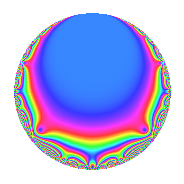# Properties

 Label 4018.2.a.xLevel 4018 Weight 2 Character orbit 4018.a Self dual Yes Analytic conductor 32.084 Analytic rank 1 Dimension 2 CM No Inner twists 1

# Related objects

## Newspace parameters

 Level: $$N$$ = $$4018 = 2 \cdot 7^{2} \cdot 41$$ Weight: $$k$$ = $$2$$ Character orbit: $$[\chi]$$ = 4018.a (trivial)

## Newform invariants

 Self dual: Yes Analytic conductor: $$32.0838915322$$ Analytic rank: $$1$$ Dimension: $$2$$ Coefficient field: $$\Q(\sqrt{3})$$ Coefficient ring: $$\Z[a_1, \ldots, a_{5}]$$ Coefficient ring index: $$1$$ Fricke sign: $$1$$ Sato-Tate group: $\mathrm{SU}(2)$

## $q$-expansion

Coefficients of the $$q$$-expansion are expressed in terms of $$\beta = \sqrt{3}$$. We also show the integral $$q$$-expansion of the trace form.

 $$f(q)$$ $$=$$ $$q$$ $$+ q^{2}$$ $$-2 q^{3}$$ $$+ q^{4}$$ $$+ ( -1 - \beta ) q^{5}$$ $$-2 q^{6}$$ $$+ q^{8}$$ $$+ q^{9}$$ $$+O(q^{10})$$ $$q$$ $$+ q^{2}$$ $$-2 q^{3}$$ $$+ q^{4}$$ $$+ ( -1 - \beta ) q^{5}$$ $$-2 q^{6}$$ $$+ q^{8}$$ $$+ q^{9}$$ $$+ ( -1 - \beta ) q^{10}$$ $$+ ( -1 - \beta ) q^{11}$$ $$-2 q^{12}$$ $$+ ( 2 + 2 \beta ) q^{13}$$ $$+ ( 2 + 2 \beta ) q^{15}$$ $$+ q^{16}$$ $$+ q^{18}$$ $$+ 2 \beta q^{19}$$ $$+ ( -1 - \beta ) q^{20}$$ $$+ ( -1 - \beta ) q^{22}$$ $$-2 q^{23}$$ $$-2 q^{24}$$ $$+ ( -1 + 2 \beta ) q^{25}$$ $$+ ( 2 + 2 \beta ) q^{26}$$ $$+ 4 q^{27}$$ $$+ ( 1 - 5 \beta ) q^{29}$$ $$+ ( 2 + 2 \beta ) q^{30}$$ $$+ ( 2 - 2 \beta ) q^{31}$$ $$+ q^{32}$$ $$+ ( 2 + 2 \beta ) q^{33}$$ $$+ q^{36}$$ $$+ ( -2 + 4 \beta ) q^{37}$$ $$+ 2 \beta q^{38}$$ $$+ ( -4 - 4 \beta ) q^{39}$$ $$+ ( -1 - \beta ) q^{40}$$ $$- q^{41}$$ $$+ ( 2 + 6 \beta ) q^{43}$$ $$+ ( -1 - \beta ) q^{44}$$ $$+ ( -1 - \beta ) q^{45}$$ $$-2 q^{46}$$ $$+ ( -4 + 2 \beta ) q^{47}$$ $$-2 q^{48}$$ $$+ ( -1 + 2 \beta ) q^{50}$$ $$+ ( 2 + 2 \beta ) q^{52}$$ $$+ ( -1 + 5 \beta ) q^{53}$$ $$+ 4 q^{54}$$ $$+ ( 4 + 2 \beta ) q^{55}$$ $$-4 \beta q^{57}$$ $$+ ( 1 - 5 \beta ) q^{58}$$ $$+ ( -1 - 7 \beta ) q^{59}$$ $$+ ( 2 + 2 \beta ) q^{60}$$ $$+ ( -5 - \beta ) q^{61}$$ $$+ ( 2 - 2 \beta ) q^{62}$$ $$+ q^{64}$$ $$+ ( -8 - 4 \beta ) q^{65}$$ $$+ ( 2 + 2 \beta ) q^{66}$$ $$+ ( -5 + 3 \beta ) q^{67}$$ $$+ 4 q^{69}$$ $$+ ( 6 - 2 \beta ) q^{71}$$ $$+ q^{72}$$ $$+ ( 2 + 4 \beta ) q^{73}$$ $$+ ( -2 + 4 \beta ) q^{74}$$ $$+ ( 2 - 4 \beta ) q^{75}$$ $$+ 2 \beta q^{76}$$ $$+ ( -4 - 4 \beta ) q^{78}$$ $$+ ( -1 - \beta ) q^{80}$$ $$-11 q^{81}$$ $$- q^{82}$$ $$+ ( -3 - \beta ) q^{83}$$ $$+ ( 2 + 6 \beta ) q^{86}$$ $$+ ( -2 + 10 \beta ) q^{87}$$ $$+ ( -1 - \beta ) q^{88}$$ $$+ ( -6 - 4 \beta ) q^{89}$$ $$+ ( -1 - \beta ) q^{90}$$ $$-2 q^{92}$$ $$+ ( -4 + 4 \beta ) q^{93}$$ $$+ ( -4 + 2 \beta ) q^{94}$$ $$+ ( -6 - 2 \beta ) q^{95}$$ $$-2 q^{96}$$ $$-2 q^{97}$$ $$+ ( -1 - \beta ) q^{99}$$ $$+O(q^{100})$$ $$\operatorname{Tr}(f)(q)$$ $$=$$ $$2q$$ $$\mathstrut +\mathstrut 2q^{2}$$ $$\mathstrut -\mathstrut 4q^{3}$$ $$\mathstrut +\mathstrut 2q^{4}$$ $$\mathstrut -\mathstrut 2q^{5}$$ $$\mathstrut -\mathstrut 4q^{6}$$ $$\mathstrut +\mathstrut 2q^{8}$$ $$\mathstrut +\mathstrut 2q^{9}$$ $$\mathstrut +\mathstrut O(q^{10})$$ $$2q$$ $$\mathstrut +\mathstrut 2q^{2}$$ $$\mathstrut -\mathstrut 4q^{3}$$ $$\mathstrut +\mathstrut 2q^{4}$$ $$\mathstrut -\mathstrut 2q^{5}$$ $$\mathstrut -\mathstrut 4q^{6}$$ $$\mathstrut +\mathstrut 2q^{8}$$ $$\mathstrut +\mathstrut 2q^{9}$$ $$\mathstrut -\mathstrut 2q^{10}$$ $$\mathstrut -\mathstrut 2q^{11}$$ $$\mathstrut -\mathstrut 4q^{12}$$ $$\mathstrut +\mathstrut 4q^{13}$$ $$\mathstrut +\mathstrut 4q^{15}$$ $$\mathstrut +\mathstrut 2q^{16}$$ $$\mathstrut +\mathstrut 2q^{18}$$ $$\mathstrut -\mathstrut 2q^{20}$$ $$\mathstrut -\mathstrut 2q^{22}$$ $$\mathstrut -\mathstrut 4q^{23}$$ $$\mathstrut -\mathstrut 4q^{24}$$ $$\mathstrut -\mathstrut 2q^{25}$$ $$\mathstrut +\mathstrut 4q^{26}$$ $$\mathstrut +\mathstrut 8q^{27}$$ $$\mathstrut +\mathstrut 2q^{29}$$ $$\mathstrut +\mathstrut 4q^{30}$$ $$\mathstrut +\mathstrut 4q^{31}$$ $$\mathstrut +\mathstrut 2q^{32}$$ $$\mathstrut +\mathstrut 4q^{33}$$ $$\mathstrut +\mathstrut 2q^{36}$$ $$\mathstrut -\mathstrut 4q^{37}$$ $$\mathstrut -\mathstrut 8q^{39}$$ $$\mathstrut -\mathstrut 2q^{40}$$ $$\mathstrut -\mathstrut 2q^{41}$$ $$\mathstrut +\mathstrut 4q^{43}$$ $$\mathstrut -\mathstrut 2q^{44}$$ $$\mathstrut -\mathstrut 2q^{45}$$ $$\mathstrut -\mathstrut 4q^{46}$$ $$\mathstrut -\mathstrut 8q^{47}$$ $$\mathstrut -\mathstrut 4q^{48}$$ $$\mathstrut -\mathstrut 2q^{50}$$ $$\mathstrut +\mathstrut 4q^{52}$$ $$\mathstrut -\mathstrut 2q^{53}$$ $$\mathstrut +\mathstrut 8q^{54}$$ $$\mathstrut +\mathstrut 8q^{55}$$ $$\mathstrut +\mathstrut 2q^{58}$$ $$\mathstrut -\mathstrut 2q^{59}$$ $$\mathstrut +\mathstrut 4q^{60}$$ $$\mathstrut -\mathstrut 10q^{61}$$ $$\mathstrut +\mathstrut 4q^{62}$$ $$\mathstrut +\mathstrut 2q^{64}$$ $$\mathstrut -\mathstrut 16q^{65}$$ $$\mathstrut +\mathstrut 4q^{66}$$ $$\mathstrut -\mathstrut 10q^{67}$$ $$\mathstrut +\mathstrut 8q^{69}$$ $$\mathstrut +\mathstrut 12q^{71}$$ $$\mathstrut +\mathstrut 2q^{72}$$ $$\mathstrut +\mathstrut 4q^{73}$$ $$\mathstrut -\mathstrut 4q^{74}$$ $$\mathstrut +\mathstrut 4q^{75}$$ $$\mathstrut -\mathstrut 8q^{78}$$ $$\mathstrut -\mathstrut 2q^{80}$$ $$\mathstrut -\mathstrut 22q^{81}$$ $$\mathstrut -\mathstrut 2q^{82}$$ $$\mathstrut -\mathstrut 6q^{83}$$ $$\mathstrut +\mathstrut 4q^{86}$$ $$\mathstrut -\mathstrut 4q^{87}$$ $$\mathstrut -\mathstrut 2q^{88}$$ $$\mathstrut -\mathstrut 12q^{89}$$ $$\mathstrut -\mathstrut 2q^{90}$$ $$\mathstrut -\mathstrut 4q^{92}$$ $$\mathstrut -\mathstrut 8q^{93}$$ $$\mathstrut -\mathstrut 8q^{94}$$ $$\mathstrut -\mathstrut 12q^{95}$$ $$\mathstrut -\mathstrut 4q^{96}$$ $$\mathstrut -\mathstrut 4q^{97}$$ $$\mathstrut -\mathstrut 2q^{99}$$ $$\mathstrut +\mathstrut O(q^{100})$$

## Embeddings

For each embedding $$\iota_m$$ of the coefficient field, the values $$\iota_m(a_n)$$ are shown below.

For more information on an embedded modular form you can click on its label.

Label $$\iota_m(\nu)$$ $$a_{2}$$ $$a_{3}$$ $$a_{4}$$ $$a_{5}$$ $$a_{6}$$ $$a_{7}$$ $$a_{8}$$ $$a_{9}$$ $$a_{10}$$
1.1
 1.73205 −1.73205
1.00000 −2.00000 1.00000 −2.73205 −2.00000 0 1.00000 1.00000 −2.73205
1.2 1.00000 −2.00000 1.00000 0.732051 −2.00000 0 1.00000 1.00000 0.732051
 $$n$$: e.g. 2-40 or 990-1000 Significant digits: Format: Complex embeddings Normalized embeddings Satake parameters Satake angles

## Inner twists

This newform does not admit any (nontrivial) inner twists.

## Atkin-Lehner signs

$$p$$ Sign
$$2$$ $$-1$$
$$7$$ $$-1$$
$$41$$ $$1$$

## Hecke kernels

This newform can be constructed as the intersection of the kernels of the following linear operators acting on $$S_{2}^{\mathrm{new}}(\Gamma_0(4018))$$:

 $$T_{3}$$ $$\mathstrut +\mathstrut 2$$ $$T_{5}^{2}$$ $$\mathstrut +\mathstrut 2 T_{5}$$ $$\mathstrut -\mathstrut 2$$ $$T_{11}^{2}$$ $$\mathstrut +\mathstrut 2 T_{11}$$ $$\mathstrut -\mathstrut 2$$